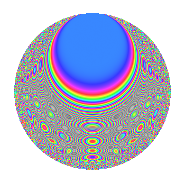# Properties

 Label 231.2.yLevel 231 Weight 2 Character orbit y Rep. character $$\chi_{231}(4,\cdot)$$ Character field $$\Q(\zeta_{15})$$ Dimension 128 Newform subspaces 2 Sturm bound 64 Trace bound 2

# Related objects

## Defining parameters

 Level: $$N$$ = $$231 = 3 \cdot 7 \cdot 11$$ Weight: $$k$$ = $$2$$ Character orbit: $$[\chi]$$ = 231.y (of order $$15$$ and degree $$8$$) Character conductor: $$\operatorname{cond}(\chi)$$ = $$77$$ Character field: $$\Q(\zeta_{15})$$ Newform subspaces: $$2$$ Sturm bound: $$64$$ Trace bound: $$2$$ Distinguishing $$T_p$$: $$2$$

## Dimensions

The following table gives the dimensions of various subspaces of $$M_{2}(231, [\chi])$$.

Total New Old
Modular forms 288 128 160
Cusp forms 224 128 96
Eisenstein series 64 0 64

## Trace form

 $$128q + 4q^{2} + 20q^{4} - 4q^{5} - 8q^{6} - 2q^{7} + 16q^{8} + 16q^{9} + O(q^{10})$$ $$128q + 4q^{2} + 20q^{4} - 4q^{5} - 8q^{6} - 2q^{7} + 16q^{8} + 16q^{9} - 36q^{10} - 2q^{11} - 16q^{13} - 20q^{14} - 12q^{15} - 6q^{18} + 8q^{19} + 48q^{20} - 52q^{22} - 8q^{23} + 30q^{24} - 12q^{25} + 8q^{26} - 38q^{28} - 56q^{29} + 8q^{30} + 6q^{31} + 8q^{32} - 6q^{33} - 144q^{34} + 36q^{35} - 40q^{36} - 32q^{37} - 8q^{38} + 42q^{40} - 48q^{41} - 2q^{42} - 8q^{43} - 26q^{44} - 4q^{45} - 38q^{46} - 44q^{47} + 66q^{49} - 56q^{50} + 12q^{51} + 12q^{52} - 52q^{53} - 16q^{54} - 56q^{55} - 24q^{56} - 16q^{57} + 12q^{58} + 8q^{59} - 18q^{61} + 96q^{62} - 14q^{63} - 96q^{64} + 16q^{65} - 12q^{66} + 72q^{67} - 56q^{68} + 24q^{69} + 58q^{70} + 128q^{71} + 2q^{72} - 6q^{73} + 4q^{74} - 8q^{75} + 272q^{76} + 4q^{77} + 120q^{78} - 6q^{79} - 88q^{80} + 16q^{81} + 128q^{83} - 12q^{84} + 172q^{85} - 34q^{86} - 100q^{87} + 28q^{88} + 72q^{89} - 8q^{90} + 300q^{92} - 44q^{93} - 52q^{94} - 10q^{95} + 4q^{96} + 32q^{97} - 240q^{98} + 44q^{99} + O(q^{100})$$

## Decomposition of $$S_{2}^{\mathrm{new}}(231, [\chi])$$ into newform subspaces

Label Dim. $$A$$ Field CM Traces $q$-expansion
$$a_2$$ $$a_3$$ $$a_5$$ $$a_7$$
231.2.y.a $$64$$ $$1.845$$ None $$0$$ $$-8$$ $$0$$ $$-1$$
231.2.y.b $$64$$ $$1.845$$ None $$4$$ $$8$$ $$-4$$ $$-1$$

## Decomposition of $$S_{2}^{\mathrm{old}}(231, [\chi])$$ into lower level spaces

$$S_{2}^{\mathrm{old}}(231, [\chi]) \cong$$ $$S_{2}^{\mathrm{new}}(77, [\chi])$$$$^{\oplus 2}$$

## Hecke Characteristic Polynomials

There are no characteristic polynomials of Hecke operators in the database